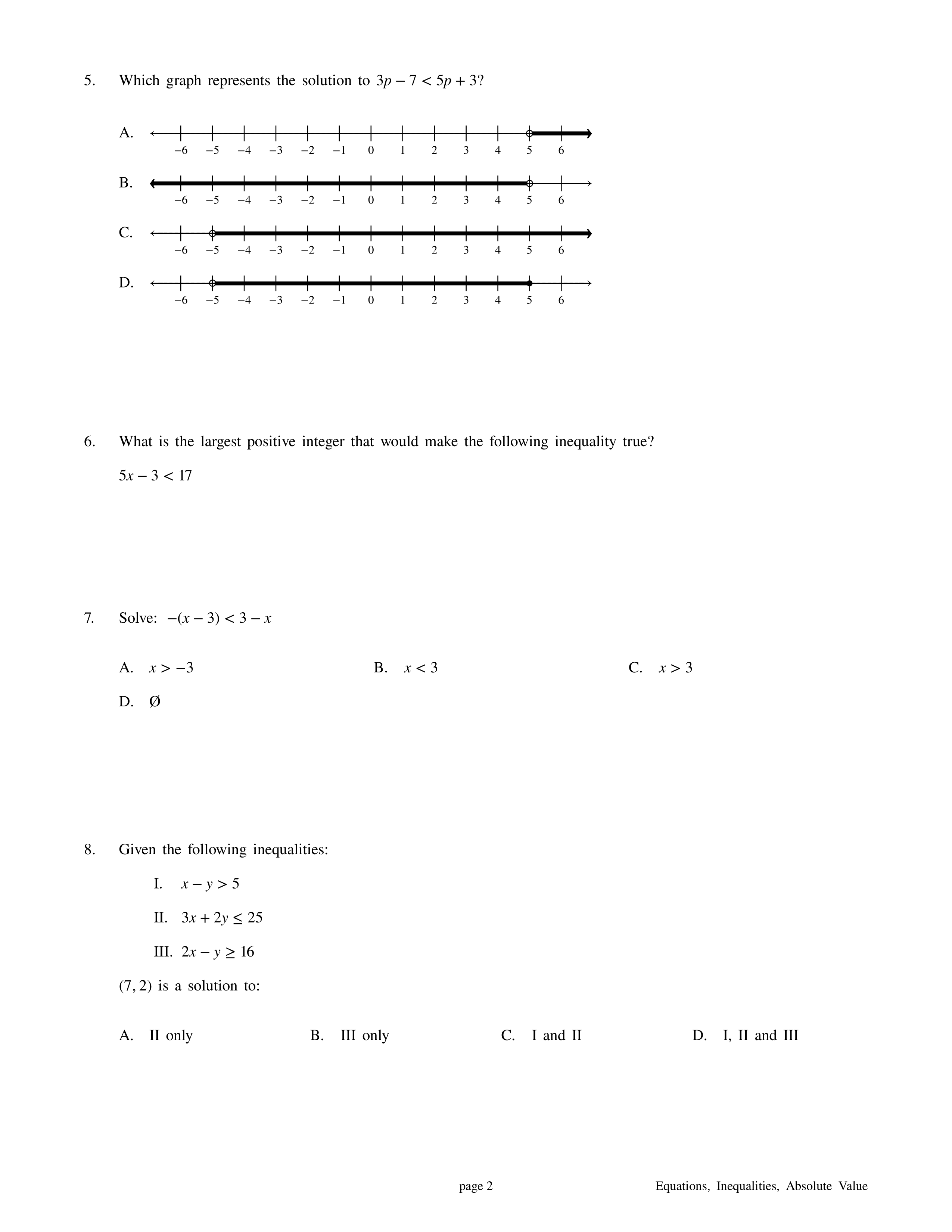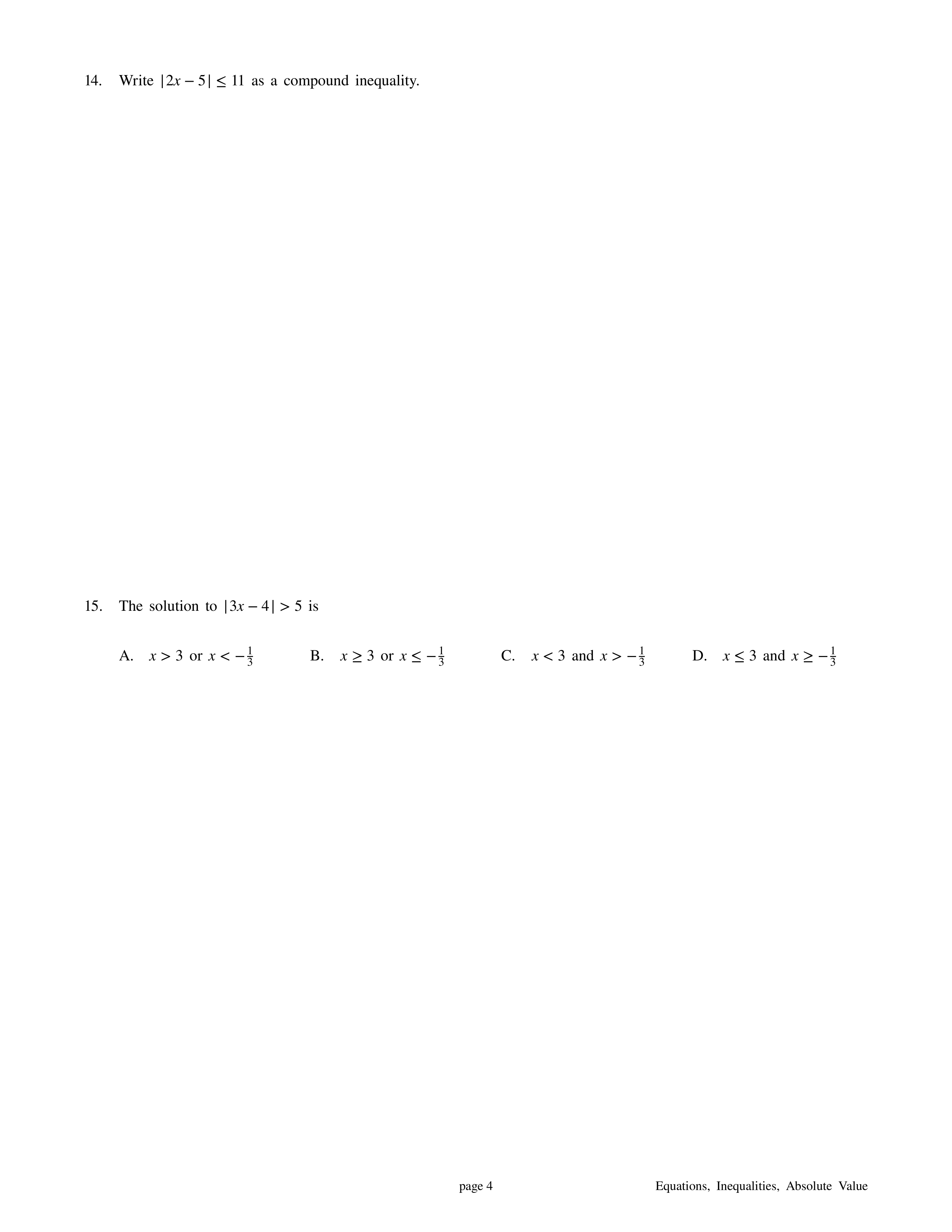Summative: Linear Equations, Inequalities, Absolute Value
starstarstarstarstarstarstarstarstarstar
by Felix Gabathuler
| 18 Questions
Standards to be assessed:

CCSS.MATH.CONTENT.7.EE.B.4.A Solve word problems leading to equations of the form px + q = r and p(x + q) = r, where p, q, and r are specific rational numbers. Solve equations of these forms fluently.

CCSS.MATH.CONTENT.7.EE.B.4.B Solve word problems leading to inequalities of the form px + q > r or px + q < r, where p, q, and r are specific rational numbers. Graph the solution set of the inequality and interpret it in the context of the problem.1
2
3
4
1
1
A
B
C
D
2
1
A
B
C
D
3
1
A
B
C
D
4
1
A
B
C
D5
6
7
8
5
1
A
B
C
D
6
1
7
1
A
B
C
D
8
1
A
B
C
D9
10
11
12
13
9
1
A
B
C
D
10
1
11
1
Write only the answer. Do not include units.
12
1
Check all that apply.
A
B
C
D
13
1
A
B
C
D14
15
16
14
1
For #14, write your compound inequality and then graph it on the provided number line. Please be neat and precise!
15
1
A
B
C
D
16
1
Graph the solution to Question # 15 on the number line provided. Please be precise and neat!
17
1
Graph the following linear equation. Make sure to be neat and precise.
18
1
Graph the following linear inequality. Make sure to be neat and precise.